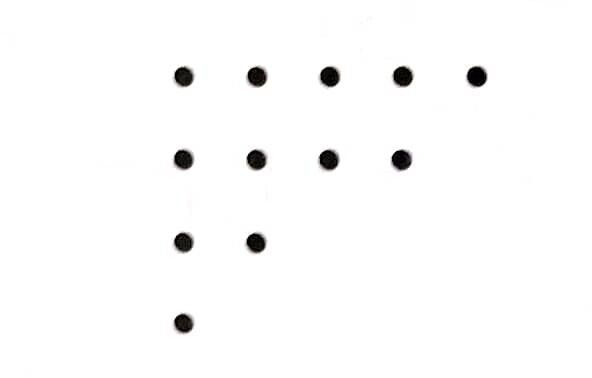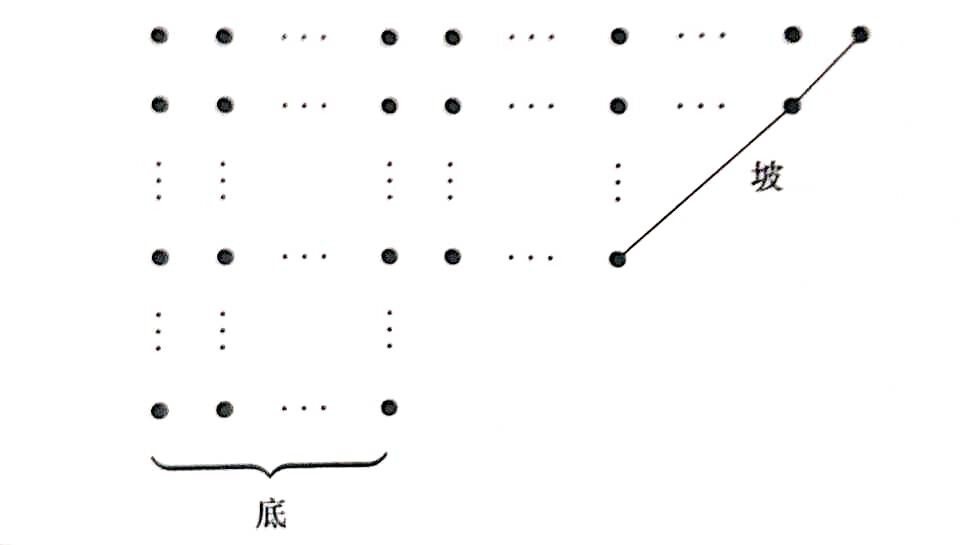# 分拆数

n012345678
112357111522

## k 部分拆数

k012345678
100000000
010000000
011000000
011100000
012110000
012211000
013321100
013432110
014553211

### 例题

  1 2 3 4 5 6 7 8 9 10 11 12 13 14 15 16 17 18 19 20 21 22 23 24 #include #include int p; /*将自然数n分拆为k个部分的方法数*/ int main() { int n, k; while (~scanf("%d%d", &n, &k)) { memset(p, 0, sizeof(p)); p = 1; int i; for (i = 1; i <= n; ++i) { int j; for (j = 1; j <= k; ++j) { if (i - j >= 0) /*p[i-j][j]所有部分大于1*/ { p[i][j] = (p[i - j][j] + p[i - 1][j - 1]) % 1000007; /*p[i-1][j-1]至少有一个部分为1。*/ } } } printf("%d\n", p[n][k]); } } 

### Ferrers 图

Ferrers 图：将分拆的每个部分用点组成的行表示。每行点的个数为这个部分的大小。## 互异分拆数

n012345678
111223456

k012345678
100000000
010000000
010000000
011000000
011000000
012000000
012100000
013100000
013200000

### 例题

  1 2 3 4 5 6 7 8 9 10 11 12 13 14 15 16 17 18 19 20 21 22 23 24 25 26 27 28 29 #include #include int pd; /*将自然数n分拆为k个部分的互异方法数*/ int main() { int n; while (~scanf("%d", &n)) { memset(pd, 0, sizeof(pd)); pd = 1; int ans = 0; int j; for (j = 1; j < 350; ++j) { int i; for (i = 0; i < 350; ++i) { pd[i][j & 1] = 0; /*pd[i][j]只与pd[][j]和pd[][j-1]有关*/ } for (i = 0; i <= n; ++i) { if (i - j >= 0) /*pd[i-j][j]所有部分大于1*/ { pd[i][j & 1] = (pd[i - j][j & 1] + pd[i - j][(j - 1) & 1]) % 1000007; /*pd[i-j][j-1]至少有一个部分为1。*/ } } ans = (ans + pd[n][j & 1]) % 1000007; } printf("%d\n", ans); } } 

## 五边形数定理### 例题

  1 2 3 4 5 6 7 8 9 10 11 12 13 14 15 16 17 18 19 20 21 22 23 24 25 26 27 28 29 30 31 32 33 34 35 36 #include long long a; long long p; int main() { p = 1; p = 1; p = 2; int i; for (i = 1; i < 50005; i++) /*递推式系数1,2,5,7,12,15,22,26...i*(3*i-1)/2,i*(3*i+1)/2*/ { a[2 * i] = i * (i * 3 - 1) / 2; /*五边形数为1,5,12,22...i*(3*i-1)/2*/ a[2 * i + 1] = i * (i * 3 + 1) / 2; } for ( i = 3; i < 50005; i++) /*p[n]=p[n-1]+p[n-2]-p[n-5]-p[n-7]+p+p-...+p[n-i*[3i-1]/2]+p[n-i*[3i+1]/2]*/ { p[i] = 0; int j; for (j = 2; a[j] <= i; j++) /*有可能为负数,式中加1000007*/ { if (j & 2) { p[i] = (p[i] + p[i - a[j]] + 1000007) % 1000007; } else { p[i] = (p[i] - p[i - a[j]] + 1000007) % 1000007; } } } int n; while (~scanf("%d", &n)) { printf("%lld\n", p[n]); } }